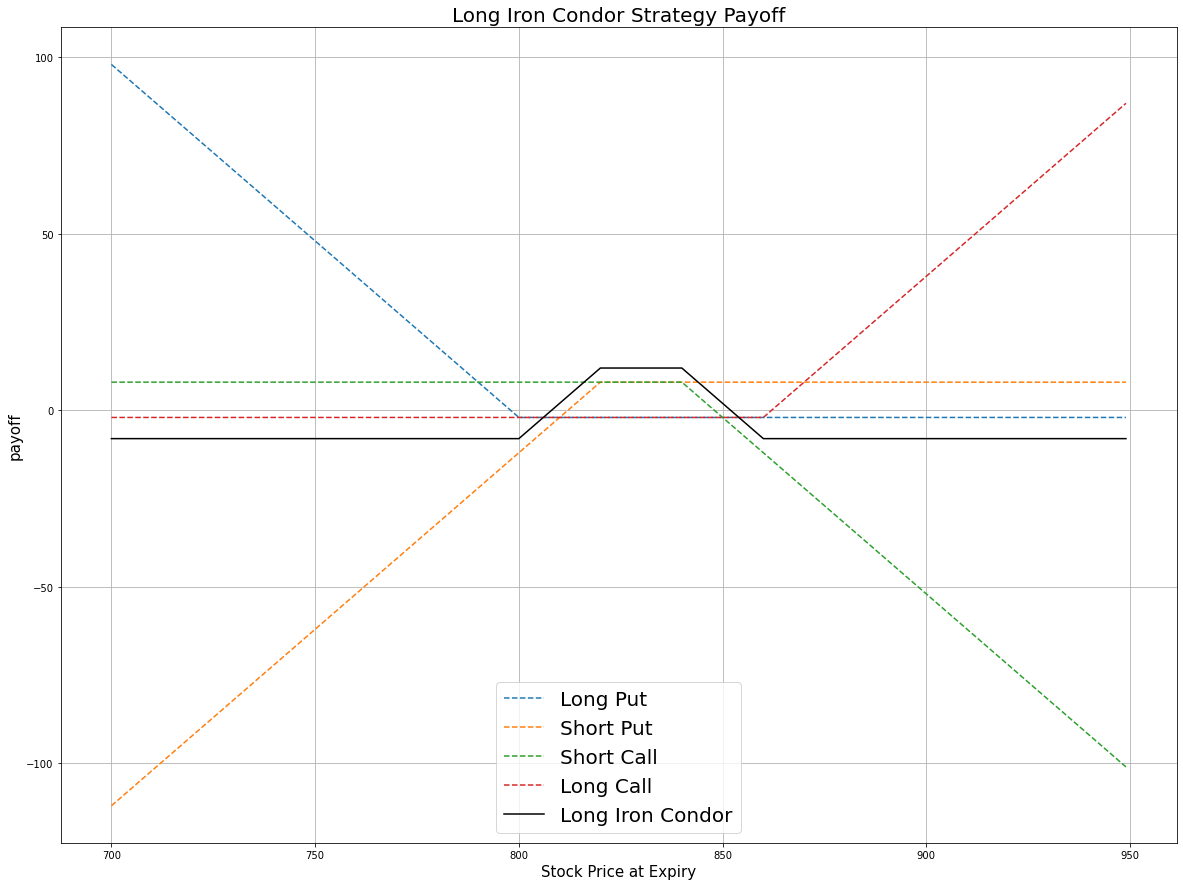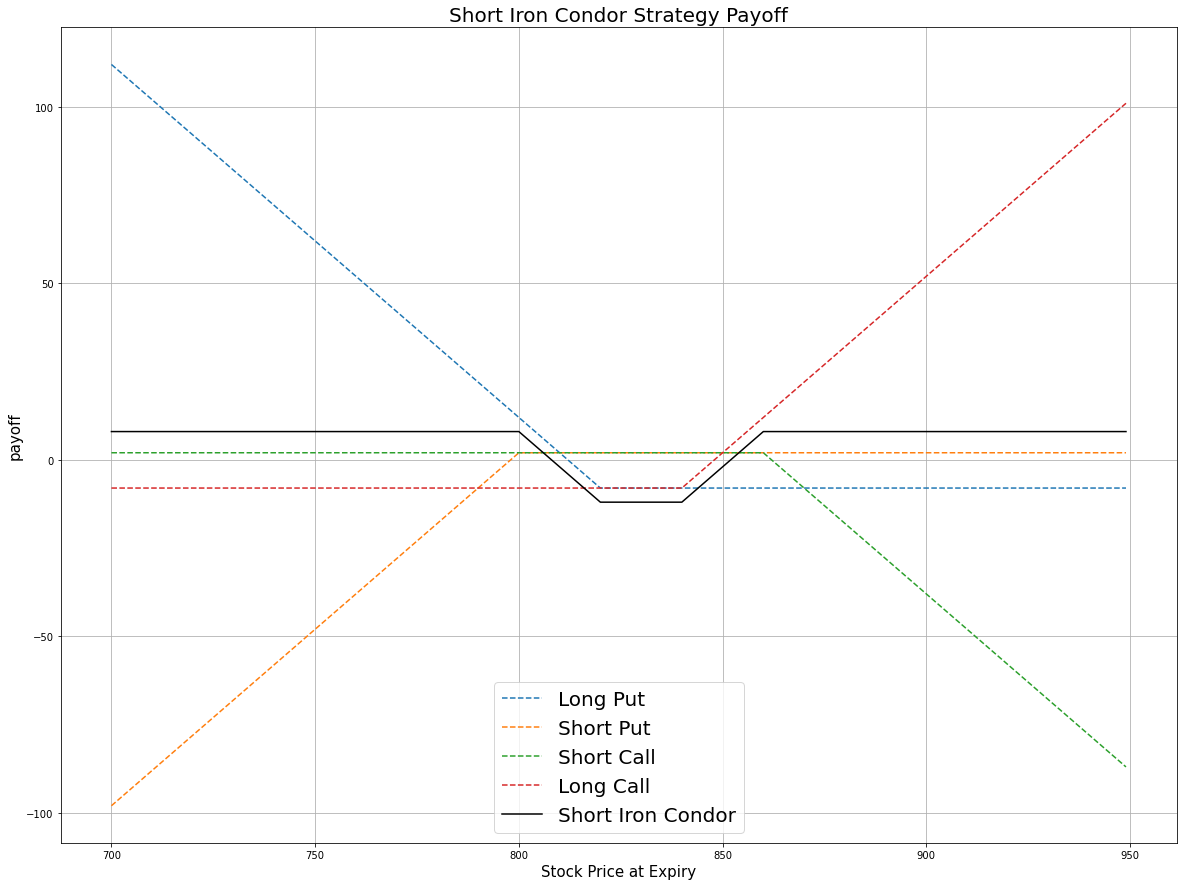# Option Strategies

## Iron Condor

### Introduction

The Iron Condor is an Option strategy that consists of four contracts. All the contracts have the same underlying Equity and expiration, but the order of strike prices is $A>B>C>D$. The following table describes the strike prices of each contract:

PositionStrike
1 far-OTM call $A$
1 near-OTM call $B, where B > underlying\ price$
1 near-OTM put $C, where C < underlying\ price$
1 far-OTM put $D, where C-D = A-B$

The iron condor can be long or short.

#### Long Iron Condor

The long iron condor consists of selling a far OTM call, selling a far OTM put, buying a near OTM call, and buying a near OTM put. This strategy profits from a increase in price movement (implied volatility).

#### Short Iron Condor

The short iron condor consists of buying a far OTM call, buying a far OTM put, selling a near ATM call, and selling a near ATM put. This strategy profits from an decrease in price movement (implied volatility) and time decay since ATM options decay sharper.

### Implementation

Follow these steps to implement the long iron condor strategy:

1. In the Initialize method, set the start date, end date, cash, and Option universe.
2. private Symbol _symbol;

public override void Initialize()
{
SetStartDate(2017, 2, 1);
SetEndDate(2017, 3, 1);
SetCash(500000);

_symbol = option.Symbol;
option.SetFilter(universe =>
universe.IncludeWeeklys().Strikes(-15, 15).Expiration(0, 40));
}
def Initialize(self) -> None:
self.SetStartDate(2017, 2, 1)
self.SetEndDate(2017, 3, 1)
self.SetCash(500000)

self.symbol = option.Symbol
option.SetFilter(lambda universe:
universe.IncludeWeeklys().Strikes(-15, 15).Expiration(0, 40))
3. In the OnData method, select the contracts in the strategy legs.
4. public override void OnData(Slice slice)
{
if (Portfolio[_symbol.Underlying].Invested)
{
Liquidate();
}

if (Portfolio.Invested || !IsMarketOpen(_symbol)) return;

if (!slice.OptionChains.TryGetValue(_symbol, out var chain)) return;

// Find put and call contracts with the farthest expiry
var expiry = chain.Max(x => x.Expiry);
var contracts = chain.Where(x => x.Expiry == expiry).OrderBy(x => x.Strike);

var putContracts = contracts.Where(x => x.Right == OptionRight.Put).ToArray();
var callContracts = contracts.Where(x => x.Right == OptionRight.Call).ToArray();

if (putContracts.Length < 10 || putContracts.Length < 10) return;

// Select the strikes in the strategy legs
var farPut = putContracts.Strike;
var nearPut = putContracts.Strike;
var nearCall = callContracts[^10].Strike;
var farCall = callContracts[^1].Strike;
def OnData(self, slice: Slice) -> None:
if self.Portfolio[self.symbol.Underlying].Invested:
self.Liquidate()

if self.Portfolio.Invested or not self.IsMarketOpen(self.symbol):
return

chain = slice.OptionChains.get(self.symbol)
if not chain:
return

# Find put and call contracts with the farthest expiry
expiry = max([x.Expiry for x in chain])
chain = sorted([x for x in chain if x.Expiry == expiry], key = lambda x: x.Strike)

put_contracts = [x for x in chain if x.Right == OptionRight.Put]
call_contracts = [x for x in chain if x.Right == OptionRight.Call]

if len(call_contracts) < 10 or len(put_contracts) < 10:
return

# Select the strikes in the strategy legs
far_put = put_contracts.Strike
near_put = put_contracts.Strike
near_call = call_contracts[-10].Strike
far_call = call_contracts[-1].Strike
5. In the OnData method, call the OptionStrategies.IronCondor method and then submit the order.
6. var ironCondor = OptionStrategies.IronCondor(
_symbol,
farPut,
nearPut,
nearCall,
nearPut,
expiry);

Buy(ironCondor, 2);
iron_condor = OptionStrategies.IronCondor(
self.symbol,
far_put,
near_put,
near_call,
far_call,
expiry)

self.Buy(iron_condor, 2)

### Strategy Payoff

The iron condor can be long or short.

#### Long Iron Condor

This is a limited-reward-limited-risk strategy. The payoff is

$$\begin{array}{rcll} C^{far}_T & = & (S_T - K^C_{far})^{+}\\ C^{near}_T & = & (S_T - K^C_{near})^{+}\\ P^{far}_T & = & (K^P_{far} - S_T)^{+}\\ P^{near}_T & = & (K^P_{near} - S_T)^{+}\\ P_T & = & (C^{near}_T + P^{near}_T - C^{far}_T - P^{far}_T - C^{near}_0 - P^{near}_0 + C^{far}_0 + P^{far}_0)\times m - fee \end{array}$$ $$\begin{array}{rcll} \textrm{where} & C^{far}_T & = & \textrm{Far OTM call value at time T}\\ & C^{near}_T & = & \textrm{Near OTM call value at time T}\\ & P^{far}_T & = & \textrm{Far OTM put value at time T}\\ & P^{near}_T & = & \textrm{Near ATM put value at time T}\\ & S_T & = & \textrm{Underlying asset price at time T}\\ & K^C_{far} & = & \textrm{Far OTM call strike price}\\ & K^C_{near} & = & \textrm{Near OTM call strike price}\\ & K^P_{far} & = & \textrm{Far OTM put strike price}\\ & K^P_{near} & = & \textrm{Near OTM put strike price}\\ & P_T & = & \textrm{Payout total at time T}\\ & C^{far}_0 & = & \textrm{Far OTM call value at position opening (credit received)}\\ & C^{near}_0 & = & \textrm{Near OTM call value at position opening (debit paid)}\\ & P^{far}_0 & = & \textrm{Far OTM put value at position opening (credit received)}\\ & P^{near}_0 & = & \textrm{Near OTM put value at position opening (debit paid)}\\ & m & = & \textrm{Contract multiplier}\\ & T & = & \textrm{Time of expiration} \end{array}$$

The following chart shows the payoff at expiration:The maximum profit is $K^C_{far} - K^C_{near} - C^{near}_0 - P^{near}_0 + C^{far}_0 + P^{far}_0$, where $K^P_{OTM} > S_T$ or $S_T > K^C_{OTM}$.

The maximum loss is the net debit paid: $C^{far}_0 + P^{far}_0 - C^{near}_0 - P^{near}_0$, where $K^P_{OTM} < S_T < K^C_{OTM}$.

If the Option is American Option, there is a risk of early assignment on the sold contracts.

#### Short Iron Condor

This is a limited-reward-limited-risk strategy. The payoff is

$$\begin{array}{rcll} C^{far}_T & = & (S_T - K^C_{far})^{+}\\ C^{near}_T & = & (S_T - K^C_{near})^{+}\\ P^{far}_T & = & (K^P_{far} - S_T)^{+}\\ P^{near}_T & = & (K^P_{near} - S_T)^{+}\\ P_T & = & (C^{far}_T + P^{far}_T - C^{near}_T - P^{near}_T - C^{far}_0 - P^{far}_0 + C^{near}_0 + P^{near}_0)\times m - fee \end{array}$$ $$\begin{array}{rcll} \textrm{where} & C^{far}_T & = & \textrm{Far OTM call value at time T}\\ & C^{near}_T & = & \textrm{Near OTM call value at time T}\\ & P^{far}_T & = & \textrm{Far OTM put value at time T}\\ & P^{near}_T & = & \textrm{Near ATM put value at time T}\\ & S_T & = & \textrm{Underlying asset price at time T}\\ & K^C_{far} & = & \textrm{Far OTM call strike price}\\ & K^C_{near} & = & \textrm{Near OTM call strike price}\\ & K^P_{far} & = & \textrm{Far OTM put strike price}\\ & K^P_{near} & = & \textrm{Near OTM put strike price}\\ & P_T & = & \textrm{Payout total at time T}\\ & C^{far}_0 & = & \textrm{Far OTM call value at position opening (credit received)}\\ & C^{near}_0 & = & \textrm{Near OTM call value at position opening (debit paid)}\\ & P^{far}_0 & = & \textrm{Far OTM put value at position opening (credit received)}\\ & P^{near}_0 & = & \textrm{Near OTM put value at position opening (debit paid)}\\ & m & = & \textrm{Contract multiplier}\\ & T & = & \textrm{Time of expiration} \end{array}$$

The following chart shows the payoff at expiration:The maximum profit is the net credit received after commission when opening the trade, where $K^P_{OTM} < S_T < K^C_{OTM}$.

The maximum loss is $K^C_{far} - K^C_{near} + C^{near}_0 + P^{near}_0 - C^{far}_0 - P^{far}_0$, where $K^P_{OTM} > S_T$ or $S_T > K^C_{OTM}$.

If the Option is American Option, there is risk of early assignment on the sold contracts.

### Example

The following table shows the price details of the assets in the algorithm:

AssetPrice ($)Strike ($)
Far-OTM call1.85857.50
Far-OTM put2.75810.00
Near-OTM call1.35855.00
Near-OTM put2.15815.00
Underlying Equity at expiration851.20-

Therefore, the payoff is

$$\begin{array}{rcll} C^{far}_T & = & (S_T - K^C_{far})^{+}\\ & = & (851.20-857.50)^{+}\\ & = & 0\\ C^{near}_T & = & (S_T - K^C_{near})^{+}\\ & = & (851.20-855.00)^{+}\\ & = & 0\\ P^{far}_T & = & (K^P_{far} - S_T)^{+}\\ & = & (815.00-851.20)^{+}\\ & = & 0\\ P^{near}_T & = & (K^P_{near} - S_T)^{+}\\ & = & (810.00-851.20)^{+}\\ & = & 0\\ P_T & = & (C^{near}_T + P^{near}_T - C^{far}_T - P^{far}_T - C^{near}_0 - P^{near}_0 + C^{far}_0 + P^{far}_0)\times m - fee\\ & = & (0+0-0-0+1.35+2.15-1.85-2.75)\times100-1\times4\\ & = & -114 \end{array}$$

So, the strategy losses \$114.

The following algorithm implements a long iron condor Option strategy:

You can also see our Videos. You can also get in touch with us via Discord.